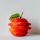Home Communities
IT Knowledge
Inspiration
Languages
EN

# Python - if statement

0 points
Created by:Khloe-Kaiser
458

In this article, we would like to show you if statement example in Python.

Quick solution:

``````x = -1

if x > 0:
print("x > 0")
elif x == 0:
print("x = 0")
else:
print("x < 0")``````

## 1. If

In this example, we create a simple `if` statement that checks if the number we specified is greater than `0`.

``````x = 1

if x > 0:
print("x > 0")``````

Output:

``x > 0``

## 2. Elif

In this example, we add `elif` keyword that checks the next condition if the previous conditions were not true.

``````x = 0

if x > 0:
print("x > 0")
elif x <= 0:
print("x <= 0")``````

Output:

``x <= 0``

## 3. Else

In this example, we add the `else` condition that catches anything which isn't caught by the previous conditions

``````x = -1

if x > 0:
print("x > 0")
elif x == 0:
print("x = 0")
else:
print("x < 0")``````

Output:

``x < 0``

## 4. Operators

You can use operators such as `and` & `or` to combine conditions inside `if` statement.

### `and` operator

``````x = 1
y = 2

if x > 0 and y > 0:
print("Both x and y are positive numbers.")``````

Output:

``Both x and y are positive numbers.``

### `or` operator

``````x = 1
y = -5

if x < 0 or y < 0:
print("One of the numbers is negative.")``````

Output:

``One of the numbers is negative.``

## Alternative titles

Join to our subscribers to be up to date with content, news and offers.